# Maths - Matrix to Euler - Sample Orientations

## Sample Rotations

In order to try to explain things and give some examples we can try I thought it might help to show the rotations for a finite subset of the rotation group. We will use the set of rotations of a cube onto itself, this is a permutation group which gives 24 possible rotations as explaned on this page.

In the following table we will need to know what quadrant the results are in, so I have taken some sample results from Math.atan2

```atan2(0.0,0.0)=0.0 (although Math.atan2 returns 0 this is abitary and if both x and y is zero we need to find some other way to get to value)
atan2(0.0,1.0)=0.0
atan2(1.0,1.0)=0.7853981633974483
atan2(1.0,0.0)=1.5707963267948966
atan2(1.0,-1.0)=2.356194490192345
atan2(0.0,-1.0)=3.141592653589793
atan2(-1.0,-1.0)=-2.356194490192345
atan2(-1.0,0.0)=-1.5707963267948966
atan2(-1.0,1.0)=-0.7853981633974483```
heading applied first giving 4 possible orientations:reference orientation

 1 0 0 0 1 0 0 0 1

attitude = asin(m10)

bank = atan2(-m12,m11)

attitude = asin(0)
bank = atan2(0,1)

attitude = 0
bank = 0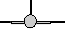rotate by 90 degrees about y axis

 0 0 1 0 1 0 -1 0 0

attitude = asin(m10)
bank = atan2(-m12,m11)

attitude = asin(0)
bank = atan2(0,1)

heading = 90 degrees
attitude = 0
bank = 0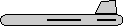rotate by 180 degrees about y axis

 -1 0 0 0 1 0 0 0 -1

attitude = asin(m10)
bank = atan2(-m12,m11)

attitude = asin(0)
bank = atan2(0,1)

heading = 180 degrees
attitude = 0
bank = 0rotate by 270 degrees about y axis

 0 0 -1 0 1 0 1 0 0

attitude = asin(m10)
bank = atan2(-m12,m11)

attitude = asin(0)
bank = atan2(0,1)

heading = -90 degrees
attitude = 0
bank = 0

Then apply attitude +90 degrees for each of the above: (note: that if we went on to apply bank to these it would just rotate between these values, the straight up and streight down orientations are known as singularities because they can be fully defined without using the bank value)0 -1 0 1 0 0 0 0 1

attitude = asin(m10)
bank = atan2(-m12,m11)

attitude = asin(1)
bank = atan2(0,0)

since M10=1 (north pole)
we use this instead:
= atan2(0,1)
bank = 0

attitude = 90 degrees
bank = 00 0 1 1 0 0 0 1 0

attitude = asin(m10)
bank = atan2(-m12,m11)

attitude = asin(1)
bank = atan2(0,0)

since M10=1 (north pole)
we use this instead:
= atan2(1,0)
bank = 0

heading = 90 degrees
attitude = 90 degrees
bank = 0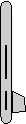0 1 0 1 0 0 0 0 -1

attitude = asin(m10)
bank = atan2(-m12,m11)

attitude = asin(1)
bank = atan2(0,0)

since M10=1 (north pole)
we use this instead:
= atan2(0,-1)
bank = 0

heading = 180 degrees
attitude = 90 degrees
bank = 00 0 -1 1 0 0 0 -1 0

attitude = asin(m10)
bank = atan2(-m12,m11)

attitude = asin(1)
bank = atan2(0,0)

since M10=1 (north pole)
we use this instead:
= atan2(-1,0)
bank = 0

heading = -90 degrees
attitude = 90 degrees
bank = 0

Or instead apply attitude -90 degrees (also a singularity):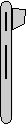0 1 0 -1 0 0 0 0 1

attitude = asin(m10)
bank = atan2(-m12,m11)

attitude = asin(-1)
bank = atan2(0,0)

since M10=-1 (south pole)
which gives:
= atan2(0,1)
bank = 0

attitude = -90 degrees
bank = 00 0 1 -1 0 0 0 -1 0

attitude = asin(m10)
bank = atan2(-m12,m11)

attitude = asin(-1)
bank = atan2(0,0)

since M10=-1 (south pole)
we use this instead:
= atan2(1,0)
bank = 0

heading = 90 degrees
attitude = -90 degrees
bank = 00 -1 0 -1 0 0 0 0 -1

attitude = asin(m10)
bank = atan2(-m12,m11)

attitude = asin(-1)
bank = atan2(0,0)

since M10=-1 (south pole)
we use this instead:
= atan2(0,-1)
bank = 0

heading = 180 degrees
attitude = -90 degrees
bank = 00 0 -1 -1 0 0 0 1 0

attitude = asin(m10)
bank = atan2(-m12,m11)

attitude = asin(-1)
bank = atan2(0,0)

since M10=-1 (south pole)
we use this instead:
= atan2(-1,0)
bank = 0

heading = -90 degrees
attitude = -90 degrees
bank = 0

Normally we dont go beond attitude + or - 90 degrees because thes are singularities, instead apply bank +90 degrees:1 0 0 0 0 -1 0 1 0

attitude = asin(m10)
bank = atan2(-m12,m11)

attitude = asin(0)
bank = atan2(1,0)

attitude = 0
bank = 90 degrees0 1 0 0 0 -1 -1 0 0

attitude = asin(m10)
bank = atan2(-m12,m11)

attitude = asin(0)
bank = atan2(1,0)

heading = 90 degrees
attitude = 0
bank = 90 degrees-1 0 0 0 0 -1 0 -1 0

attitude = asin(m10)
bank = atan2(-m12,m11)

attitude = asin(0)
bank = atan2(1,0)

heading = 180 degrees
attitude = 0
bank = 90 degrees0 -1 0 0 0 -1 1 0 0

attitude = asin(m10)
bank = atan2(-m12,m11)

attitude = asin(0)
bank = atan2(1,0)

heading = -90 degrees
attitude = 0
bank = 90 degrees

Apply bank +180 degrees:1 0 0 0 -1 0 0 0 -1

attitude = asin(m10)
bank = atan2(-m12,m11)

attitude = asin(0)
bank = atan2(0,-1)

attitude = 0
bank = 180 degrees0 0 -1 0 -1 0 -1 0 0

attitude = asin(m10)
bank = atan2(-m12,m11)

attitude = asin(0)
bank = atan2(0,-1)

heading = 90 degrees
attitude = 0
bank = 180 degrees-1 0 0 0 -1 0 0 0 1

attitude = asin(m10)
bank = atan2(-m12,m11)

attitude = asin(0)
bank = atan2(0,-1)

heading = 180 degrees
attitude = 0
bank = 180 degrees0 0 1 0 -1 0 1 0 0

attitude = asin(m10)
bank = atan2(-m12,m11)

attitude = asin(0)
bank = atan2(0,-1)

heading = -90 degrees
attitude = 0
bank = 180 degrees

Apply bank -90 degrees:1 0 0 0 0 1 0 -1 0

attitude = asin(m10)
b ank = atan2(-m12,m11)

attitude = asin(0)
bank = atan2(-1,0)

attitude = 0
bank = -90 degrees0 -1 0 0 0 1 -1 0 0

attitude = asin(m10)
bank = atan2(-m12,m11)

attitude = asin(0)
bank = atan2(-1,0)

heading = 90 degrees
attitude = 0
bank = -90 degrees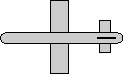-1 0 0 0 0 1 0 1 0

attitude = asin(m10)
bank = atan2(-m12,m11)

attitude = asin(0)
bank = atan2(-1,0)

heading = 180 degrees
attitude = 0
bank = -90 degrees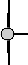0 1 0 0 0 1 1 0 0

attitude = asin(m10)
bank = atan2(-m12,m11)

attitude = asin(0)
bank = atan2(-1,0)

heading = -90 degrees
attitude = 0
bank = -90 degrees